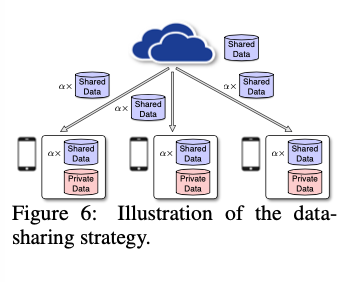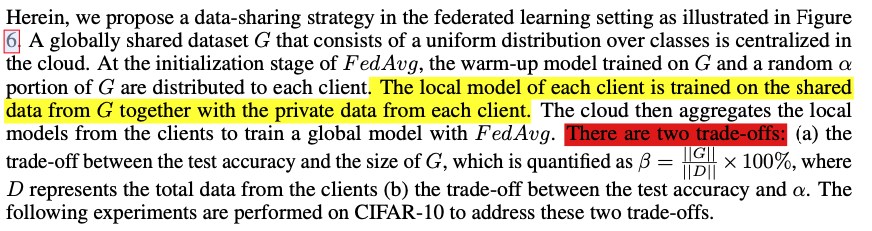## 0x01 Introduction

• IID数据比较容易训练：

The IID sampling of the training data is important to ensure that the stochastic gradient is an unbiased estimate of the full gradient

## 0x02 FedAvg on Non-IID data

### Setup

• IID：each client is randomly assigned a uniform distribution over 10 classes.
• Non-IID
• 1-class non-IID, where each client receives data partition from only a single class
• 2-class non-IID, where the sorted data is divided into 20 partitions and each client is randomly assigned 2 partitions from 2 classes.

FedAvg算法参数：

B, the batch size and E, the number of local epochs. The following parameters are used for F edAvg:

for MNIST, B = 10 and 100, E = 1 and 5, η = 0.01 and decay rate = 0.995;

for CIFAR-10, B = 10 and 100, E = 1 and 5, η = 0.1 and decay rate = 0.992;

for KWS, B = 10 and 50, E = 1 and 5, η = 0.05 and decay rate = 0.992.

### Results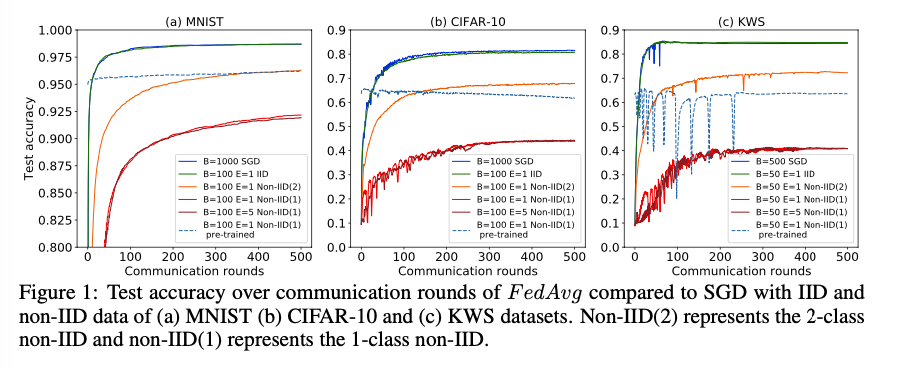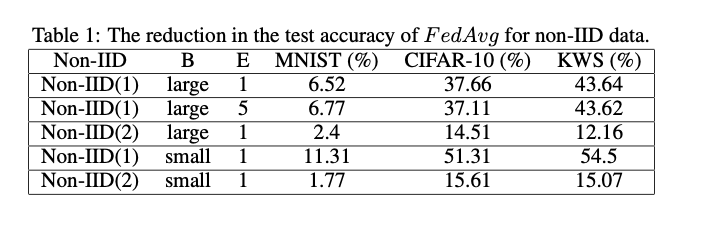## 0x03 Weight Divergence due to Non-IID Data

$weight\ divergence =||w^{FedAvg}-w^{SGD}||/||w^{SGD}||$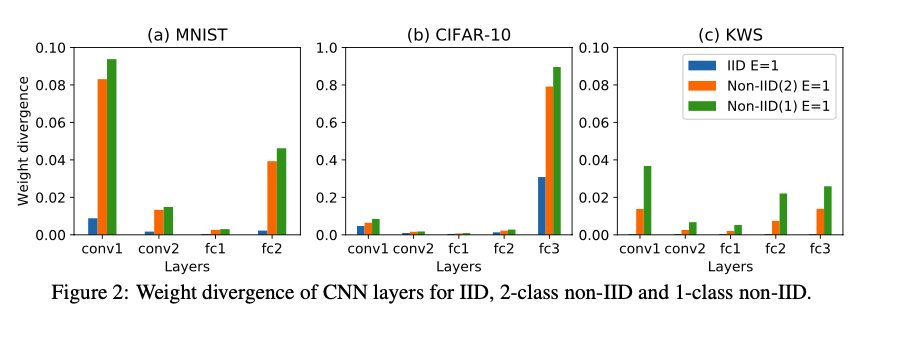## 0x04 Proposed Solution

The local model of each client is trained on the shared data from G together with the private data from each client.